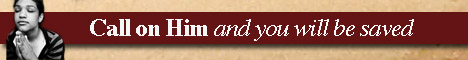Note:  Do not rely on this information. It is very old.

# Exponents

Exponents, in algebra, are the indices or powers to which various quantities are raised. Thus if a quantity x is to be raised to the seventh power it is written x7, the 7 being the exponent equal bulk has been computed as follows: -

The theory of indices shows the general extension of such exponents to fractional and negative values. [Indices.]